# Class 12 RD Sharma Solutions – Chapter 30 Linear Programming – Exercise 30.2 | Set 3

• Last Updated : 20 May, 2021

### Question 21: Maximize Z = 3x + 3y, if possible,

Subject to the constraints

x − y ≤ 1

Attention reader! All those who say programming isn't for kids, just haven't met the right mentors yet. Join the  Demo Class for First Step to Coding Coursespecifically designed for students of class 8 to 12.

The students will get to learn more about the world of programming in these free classes which will definitely help them in making a wise career choice in the future.

x + y ≥ 3

x, y ≥ 0

Solution:

Convert the given in equations into equations, we will get the following equations:

x − y = 1, x + y = 3, x = 0 and y = 0

The area shown by x − y ≤ 1:

The line x − y = 1 coincide the coordinate axes at A(1, 0) and B(0, −1) respectively.

After connecting these points we will get the line x − y = 1.

Thus,

(0,0) assure the in equation x + y ≤ 8.

Thus,

The area in xy plane which have the origin shown the solution set of the in equation x − y ≤ 1.

The area shown by x + y ≥ 3:

The line x + y = 3 coincied the coordinate axes at C(3, 0) and D(0, 3) respectively.

After connecting these points we will get the line x + y = 3.

Thus,

(0,0) assure the in equation x + y ≥ 3.

Thus,

The area in xy plane which does not have the origin show the solution set of the in equation x + y ≥ 3.

The area shown by x ≥ 0 and y ≥ 0:

Hence,

All the points in the first quadrant assure these in equations. Thus, the first quadrant is the area shown by the in equations

x ≥ 0 and y ≥ 0.

The suitable area determined by the system of constraints x − y ≤ 1, x + y ≥ 3, x ≥ 0 and y ≥ 0 are as follows.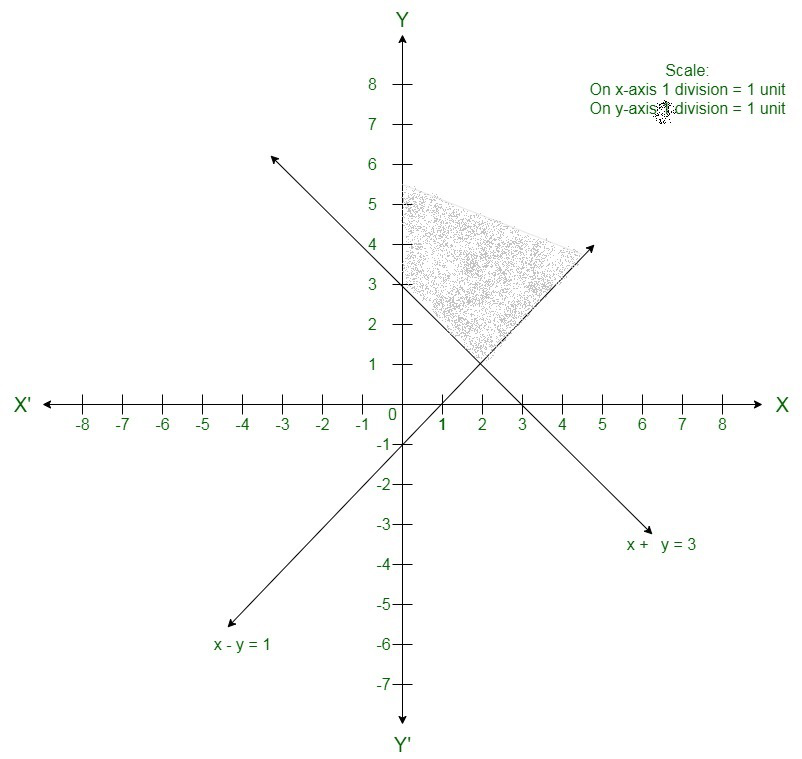The suitable area is unbounded.

We will get the maximum value at infinity.

Hence,

Maximum value will be infinity i.e. the solution is unbounded.

5x + y ≥ 10

x + y ≥ 6

x + 4y ≥ 12

x ≥ 0,

y ≥ 0

### Find x and y for which 3x + 2y is minimum subject to these inequalities. Use a graphical method.

Solution:

Convert the given in equations into equations, we will get the following equations:

5x + y = 10, x +y = 6, x + 4y = 12, x = 0 and y = 0

The area shown by 5x + y ≥ 10:

The line 5x + y = 10  coincide the coordinate axes at A(2, 0) and B(0, 10) respectively.

After connecting these points we will get the line 5x + y = 10.

Thus,

(0,0) does not assure the in equation 5x + y ≥ 10.

Thus,

The area in xy plane which does not have the origin shows the solution set of the in equation 5x + y ≥ 10.

The area shown by x +y  ≥ 6:

The line x +y = 6 coincide the coordinate axes at C(6,0) and D(0, 6) respectively.

After connecting these points we will get the line 2x +3y = 30.

Thus,

(0,0) does not assure the in equation x +y  ≥ 6.

Thus,

The area which does not have the origin shows the solution set of the in equation 2x +3y ≥ 30.

The area shown by x + 4y ≥ 12

The line x + 4y = 12 coincide the coordinate axes at E(12, 0) and F(0, 3) respectively.

After connecting these points we will get the line x + 4y = 12.

Thus,

(0,0) does not assure the in equation x + 4y ≥ 12.

Thus,

The area which does not have the origin shows the solution set of the in equation x + 4y ≥  12.

The area shown by x ≥ 0 and y ≥ 0:

Hence,

All the points in the first quadrant assure these in equations. Thus, the first quadrant is the area shown by the inequations

x ≥ 0, and y ≥ 0.

The suitable area determined by the system of constraints 5x + y ≥ 10, x +y  ≥ 6,x + 4y ≥ 12, x ≥ 0, and y ≥ 0, are as follows.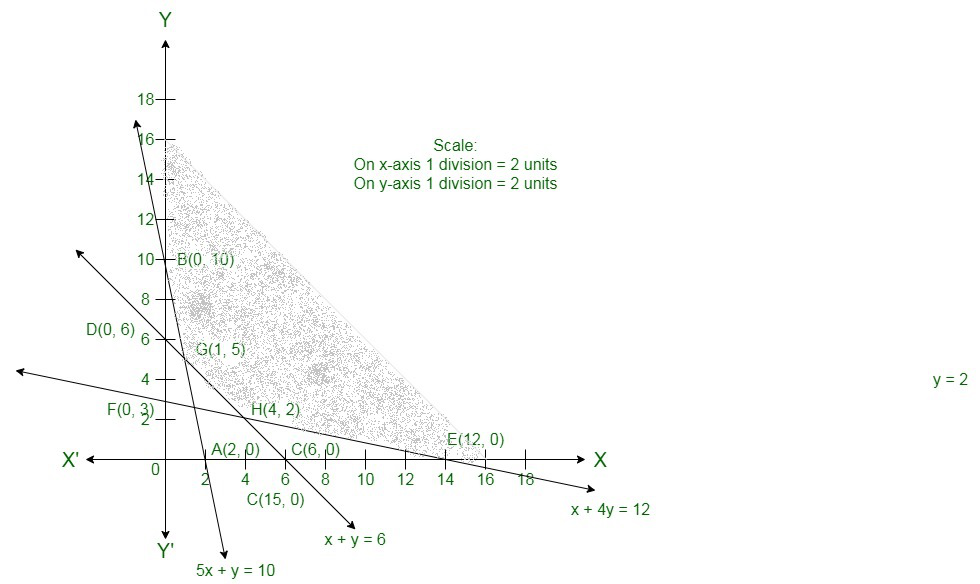The corner points of the suitable area are B(0, 10), G(1,5), H(4,2) and E(12,0).

The values of Z at these corner points are as follows.

Hence,

The minimum value of Z is 13 at the point G(1,5).

Therefore,

x = 1 and y = 5 is the best solution of the given LPP.

Therefore, the best value of Z is 13.

### Question 23: Find the maximum and minimum value of 2x + y subject to the constraints:

x + 3y ≥ 6,

x − 3y ≤ 3,

3x + 4y ≤ 24,

− 3x + 2y ≤ 6,

5x + y ≥ 5,

x, y ≥ 0.

Solution:

Convert the given in equations into equations, we will get the following equations:

x + y = 4, x + y = 3, x − 2y = 2, x = 0 and y = 0.

The line x + 3y = 6 coincide the coordinate axis at A(6, 0)A6, 0 and B(0, 2).

Connect these points to get the line x + 3y = 6.

Thus,

(0, 0) does not assure the in equation x + 3y ≥ 6. Thus, the area in xy-plane that does not have the origin represents the

solution set of the given equation.

The line x − 3y = 3 coincide the coordinate axis at C(3, 0) and D(0, −1).

Connect these points to get the line x − 3y = 3.

Thus,

(0, 0) assure the in equation x − 3y ≤ 3. Thus, the area in xy-plane that have the origin represents the solution set of the given

equation.

The line 3x + 4y = 24 meets the coordinate axis at E(8, 0) and F(0, 6). Join these points to get the line 3x + 4y = 24.

Thus,

(0, 0) assure the in equation 3x + 4y ≤ 24. Thus, the area in xy-plane that have the origin shows the solution set of the given

equation.

The line −3x + 2y = 6 coincide the coordinate axis at G(−2, 0) and H(0, 3).

Connect these points to get the line −3x + 2y = 6.

Thus, (0, 0) asuure the in equation −3x + 2y ≤ 6.

Thus,

The area in xy-plane that have the origin represents the solution set of the given equation.

The line 5x + y = 5 coincide the coordinate axis at I(1, 0)I1, 0 and J(0, 5).

Connect these points to get the line 5x + y = 5.

Thus,

(0, 0) does not assure the in equation 5x + y ≥ 5. Thus, the area in xy-plane that does not have the origin have the

solution set of the given equation.

The area shown by x ≥ 0 and y ≥ 0:

Hence,

All the points in the first quadrant assure these inequations. Thus, the first quadrant is the area shown by the in equations.

These lines are drawn using a satisfactory scale.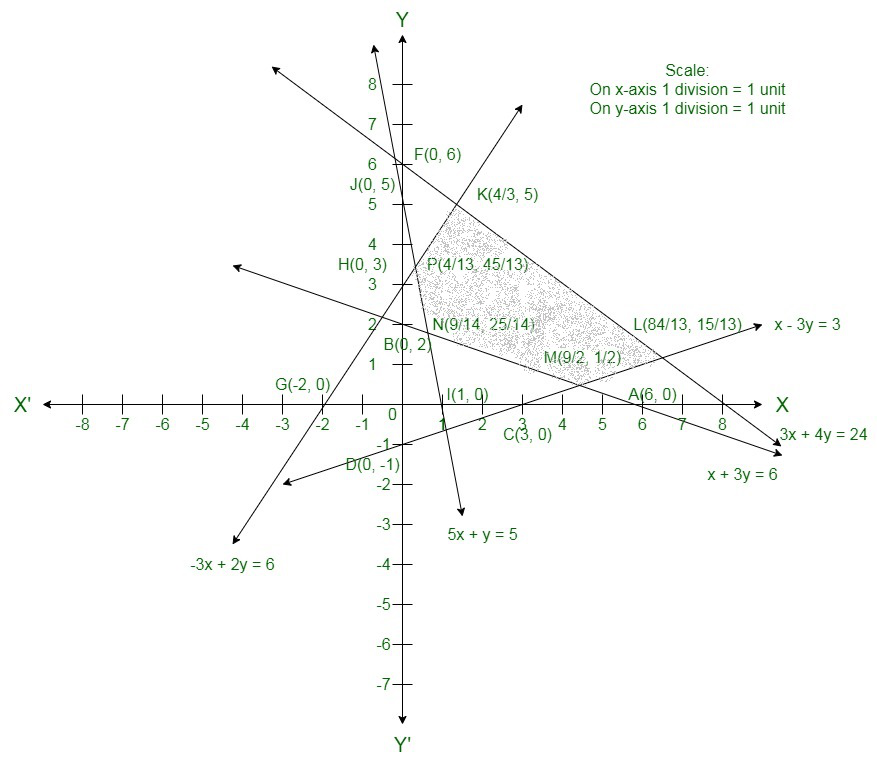The corner points of the suitable area

are P, K, L, M,The values of Z at these corner points are as follows.

Here, we can see that the minimum value of the objective function Z iswhich is at Nand maximum value of the objective function iswhich is at L.

### Question 24: Find the minimum value of 3x + 5y subject to the constraints

− 2x + y ≤ 4,

x + y ≥ 3,

x − 2y ≤ 2,

x, y ≥ 0.

Solution:

Convert the given in equations into equations, we will get the following equations:

−2x + y = 4, x + y = 3, x − 2y = 2, x = 0 and y = 0.

The line −2x + y = 4 coincide the coordinate axis at A(−2, 0)A-2, 0 and B(0, 4).

Connect these points to obtain the line −2x + y = 4.

Thus, (0, 0) assure the in equation −2x + y ≤ 4. So, the area in xy-plane that have the origin represents the solution set of the

given equation.

The line x + y = 3 coincide the coordinate axis at C(3, 0) and D(0, 3).

Connect these points to get the line x + y = 3.

Thus,

(0, 0) does not assure the in equation x + y ≥ 3. Thus, the area in xy-plane that does not have the origin shows the solution set

of the given equation.

The line x − 2y = 2 coincide the coordinate axis at E(2, 0) and F(0, −1).

Connect these points to get the line x − 2y = 2.

Thus,

(0, 0) assure the in equation x − 2y ≤ 2. Thus, the area in xy-plane that have the origin represents the solution set of the given

equation.

The area shown by x ≥ 0 and y ≥ 0:

Hence,

All the points in the first quadrant assure these in equations. Thus, the first quadrant is the area shown by the in equations.

These lines are drawn using a satisfactory scale.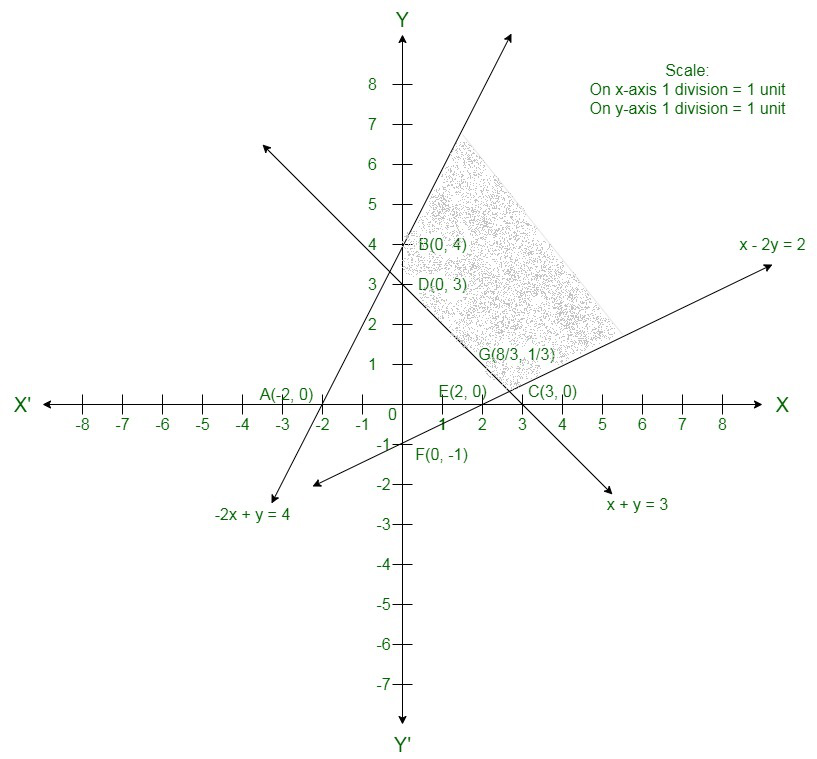The corner points of the suitable area are B(0, 4), D(0, 3) and G.

The values of Z at these corner points are as follows.

Here we can see that the minimum value of the objective function Z iswhich is at G.

Therefore,

The best value of Z is.

### Question 25: Solved the following linear programming problem graphically:

Maximize Z = 60x + 15y

Subject to constraints

x + y ≤ 50

3x + y ≤ 90

x, y≥0

Solution:

Here we have to maximize Z = 60x + 15y

Convert the given in equations into equations, we get the following equations:

x + y = 50, 3x + y = 90, x = 0 and y = 0

The area shown by x + y  ≤ 50:

The line x + y  = 50 coincide the coordinate axes at A(50,0) and B(0,50) respectively.

After connecting these points we will get line 3x + 5y = 15.

Thus,

(0,0) assure the in equation x + y  ≤ 50. Thus, the area having the origin shows the solution set of the in equation x + y  ≤ 50.

The area shown by 3x + y ≤ 90:

The line 3x + y = 90 coincide the coordinate axes at C(30, 0) and D(0, 90) respectively.

After connecting these points we will get the line 3x + y = 90.

Thus,

(0,0) assure the in equation 3x + y ≤ 90. Thus, the area having the origin shows the solution set of the in equation 3x + y ≤ 90.

The area shown by x ≥ 0 and y ≥ 0:

Hence,

All the points in the first quadrant assure these in equations. Thus, the first quadrant is the area shown by the in equations

x ≥ 0, and y ≥ 0.

The suitable area determined by the system of constraints, x + y  ≤ 50, 3x + y ≤ 90, x ≥ 0, and y ≥ 0, are as follows.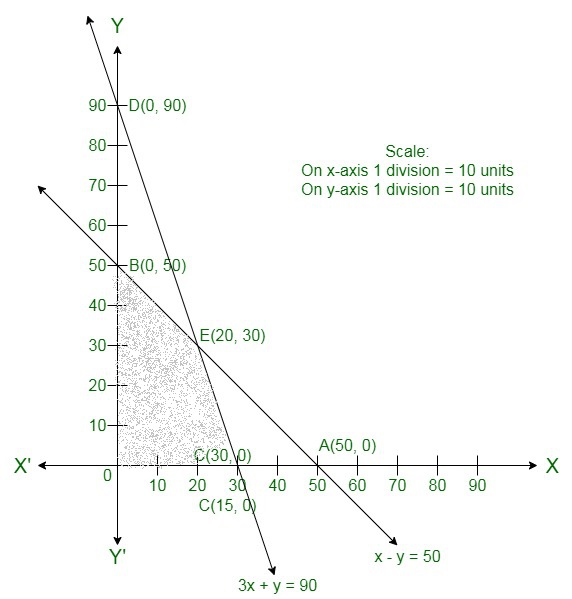The corner points of the suitable area are O(0, 0), C(30, 0), E(20, 30) and B(0, 50).

The values of Z at these corner points are as follows.

Hence,

The maximum value of Z is 1800 at the point (30, 0) at the point 30, 0.

Therefore,

x = 30 and y = 0 is the best solution of the given LPP.

Therefore,

The best value of Z is 1800.

### Question 26. Find graphically, the maximum value of Z = 2x + 5y, subject to constraints given below:

2x + 4y ≤ 8

3x + y ≤ 6

x + y ≤ 4

x ≥ 0, y ≥ 0                      [CBSE 2015]

Solution:

Here,

Maximize Z = 2x + 5y subject to the constraints

2x + 4y ≤ 8

3x + y ≤ 6

x + y ≤ 4

x ≥ 0, y ≥ 0

After converting the in equations into equations, we will get the following equations of straight lines:

2x + 4y = 8, 3x + y = 6, x + y = 4

The line 2x + 4y = 8 coincide the coordinate axes at (4, 0) and (0, 2).

The line 3x + y = 6 coincide the coordinate axes at (2, 0) and (0, 6).

The line x + y = 4 coincide the coordinate axes at (4, 0) and (0, 4).

The suitable area determined by the given constraints can be diagrammatically shown as,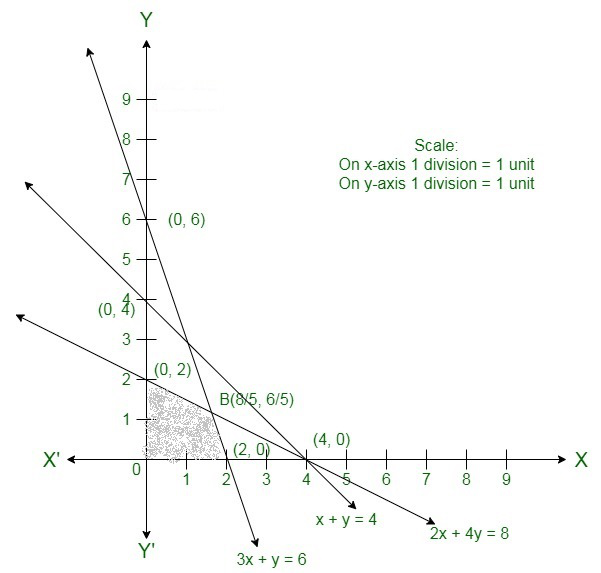The coordinates of the corner points of the suitable area are O(0, 0), A(0, 2), Band C(2, 0).

The value of the objective function at these points are given in the following table.

Therefore,

The maximum value of Z is 10 at x = 0, y = 2.

### Question 27: Solve the following LPP graphically:

Maximize Z = 20x + x + 10y

Subject to the following constraints

x + 2y ≤ 28

3x + y ≤ 24

x ≥ 2

x,y ≥  0

Solution:

Here given constraints are

x + 2y ≤ 28

3x + y ≤ 24

x ≥ 2

x, y ≥ 0

Converting the given in equations into equations,

we will get

x + 2y = 28, 3x + y = 24, x = 2, x = 0 and y = 0

These lines are drawn on the graph and the shaded region ABCD shown the suitable area of the given LPP.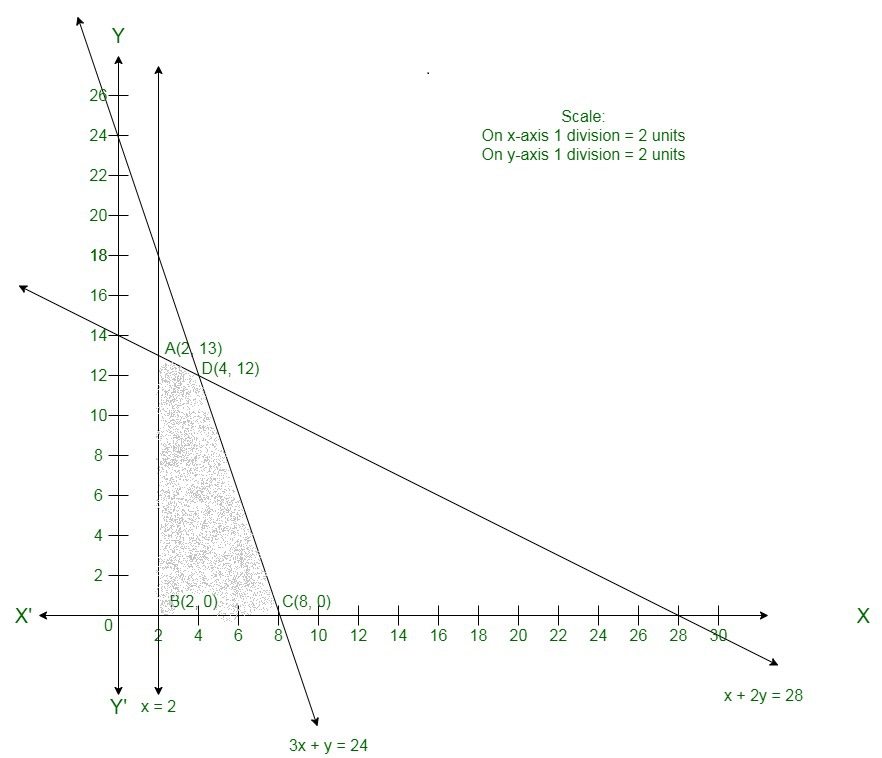It can be seen that the suitable area is bounded.

The coordinates of the corner points of the suitable area are A(2, 13), B(2, 0), C(4, 12) and D(8, 0).

The values of the objective function, Z at these corner points are given in the following table:

As can be seen in the table, is maximum at = 4 and y = 12 and the maximum value of is 200.

Therefore,

The maximum value of Z is 200.

### Question 28: Solve the following linear programming problem graphically:

Minimize z= 6x + 3y

Subject to the constraints:

4x + y ≥ 80

x + 5y ≥ 115

3x + 2y ≤ 150

x ≥ 0, y ≥ 0

Solution:

Here the given constraints are

4x + y ≥ 80

x + 5y ≥ 115

3x + 2y ≤ 150

x, y ≥ 0

Converting the given in equations into equations,

We will get

4x + y = 80, x + 5y = 115, 3x + 2y = 150, x = 0 and y = 0

These lines are drawn on the graph and the shaded area ABC represents the suitable area of the given LPP.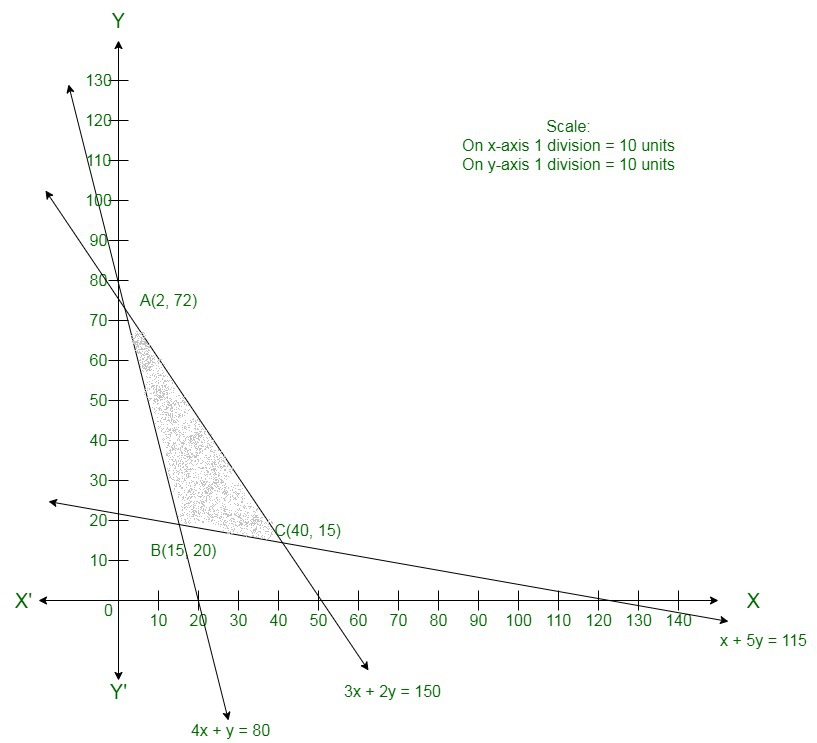It can be seen that the suitable area is bounded.

The coordinates of the corner points of the suitable are A(2, 72), B(15, 20) and C(40, 15).

The values of the objective function, Z at these corner points are given in the following table:

As seen in the table, is minimum at = 15 and y = 20 and the minimum value of is 150.

Therefore,

The minimum value of Z is 150.

My Personal Notes arrow_drop_up